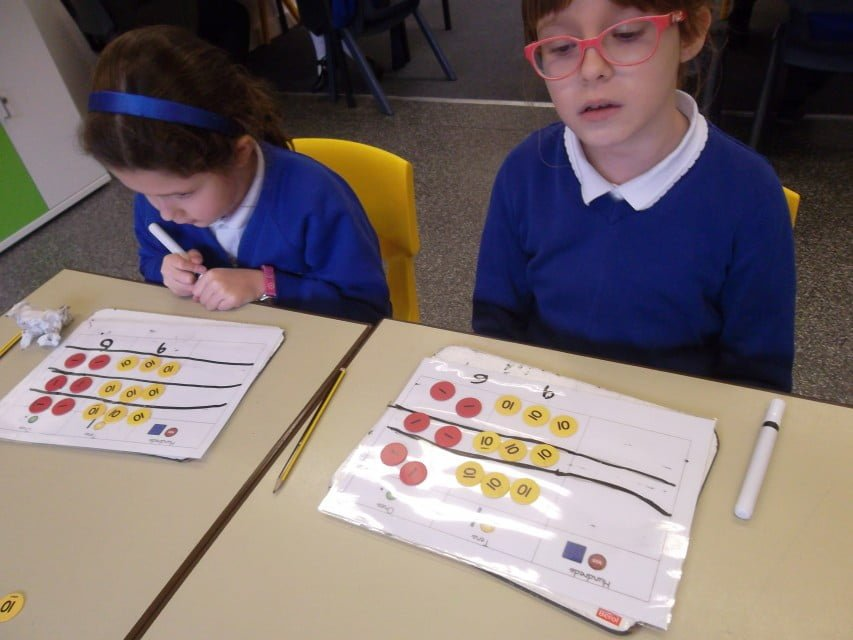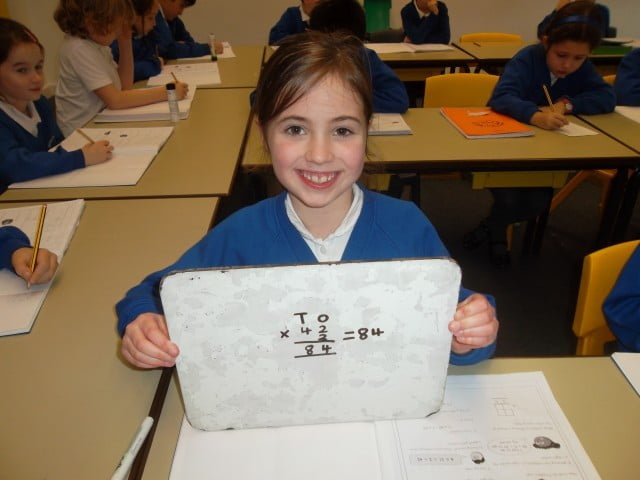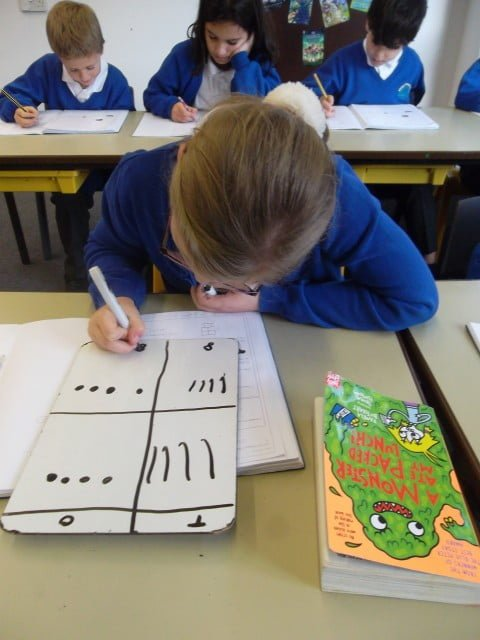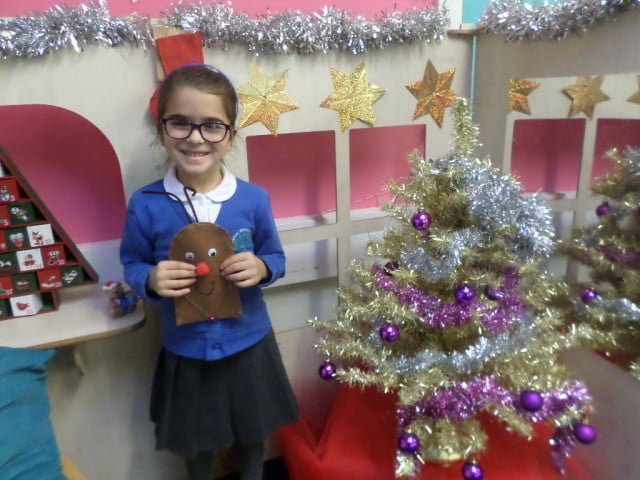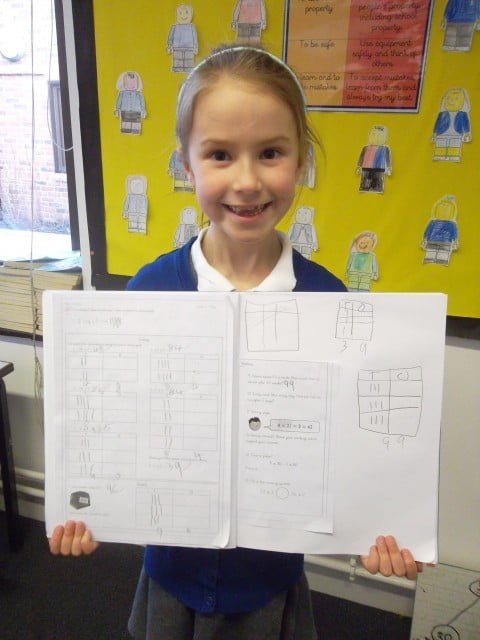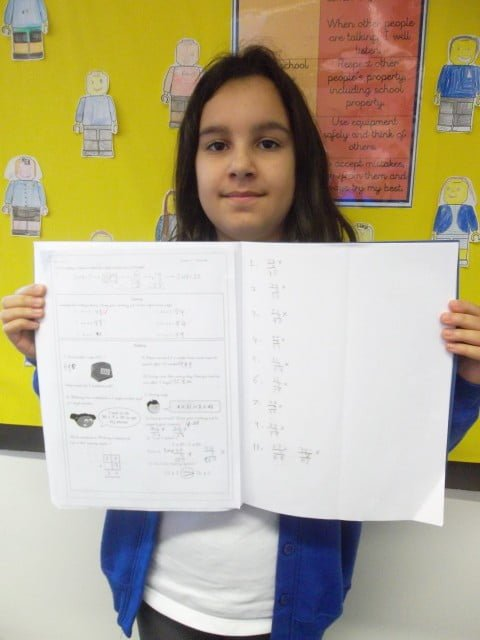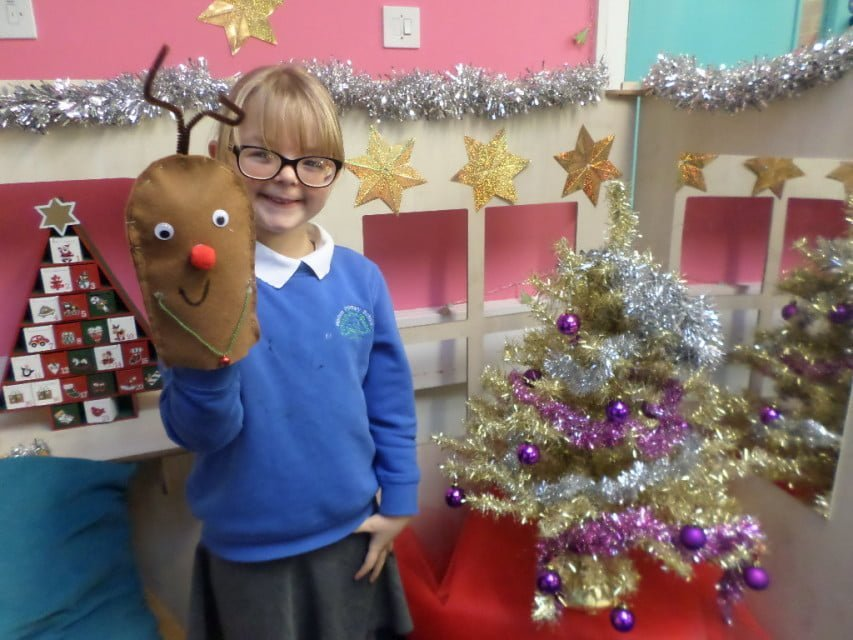# Multiplication

In maths this half term, Year 3 will be developing their multiplication and division skills. To begin with, they will be focussing on multiplication, eventually learning how to multiply any 2-digit with a 1-digit number. Their first 2 lessons introduced them to a variety of methods that they can choose from, depending on their level of understanding. They also began completing calculations that multiplied a 2-digit number by a 1-digit number without needing to exchange or regroup. In their first lesson they completed calculations practically with the place value counters before moving on to using pictorial representations of their calculation. And some children developed their learning even further by applying their understanding to different written methods. Take a look at some of the brilliant work that they completed: